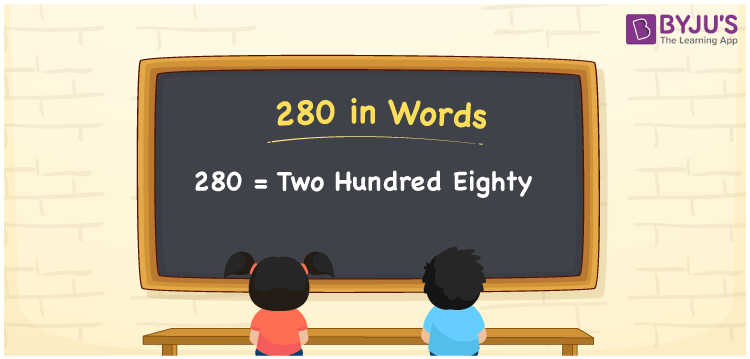# 280 in Words

280 in words is written as Two Hundred Eighty. The name of number 280 in English is “Two Hundred Eighty”. The word Two Hundred Eighty is used in many real-life situations. For example, there are Two Hundred Eighty people in a church. Thus, it is a counting number. Also, to represent the currency equal to 280, we can write it in words as Rupees Two Hundred Eighty or Two Hundred Eighty rupees. Therefore, it is necessary to learn the numbers in words, for the ease of understanding and expressing them.

 280 in words Two Hundred Eighty Two Hundred Eighty in Numbers 280

## 280 in English Words## How to Write 280 in Words?

If we know the place value of digits of 280, then we can easily express it in words. The place value is basically the position of a digit in a number. 280 is a three-digit number, therefore, we can specify the position of each digit of 280 in a place value chart. In the Indian numbering system, the order of place value of digits from right to left is given by:

 Hundreds Tens Ones 2 8 0

We can write it in expanded form as:

2 × Hundred + 8× Ten + 0 × One

= 2 × 100 + 8 × 10 + 0 × 1

= 200 + 80

= 280

= Two Hundred Eighty

Therefore, 280 in words is written as Two Hundred Eighty.

280 is a natural number that precedes 281 and succeeds 279.

280 in words – Two Hundred Eighty

Is 280 an odd number? – No

Is 280 an even number? – Yes

Is 280 a perfect square number? – No

Is 280 a perfect cube number? – No

Is 280 a prime number? – No

Is 280 a composite number? – Yes

## Frequently Asked Questions on 280 in Words

Q1

### How to write 280 in words?

280 in English is written as “Two Hundred Eighty”.
Q2

### Is 280 an odd number or even number?

280 is an even number because it is wholly divisible by 2.
280/2 = 140
Q3

### Write Two Hundred Eighty in numbers.

Two Hundred Eighty in numbers is 280.